# Machine Learning Spark machine learning Spark Mllib Machine

• Slides: 48Machine Learning • Spark machine learning – Spark Mllib • Machine learning – Supervised learning – Unsupervised learning • Regression, logistic regression, SVM • Clustering • Streaming ML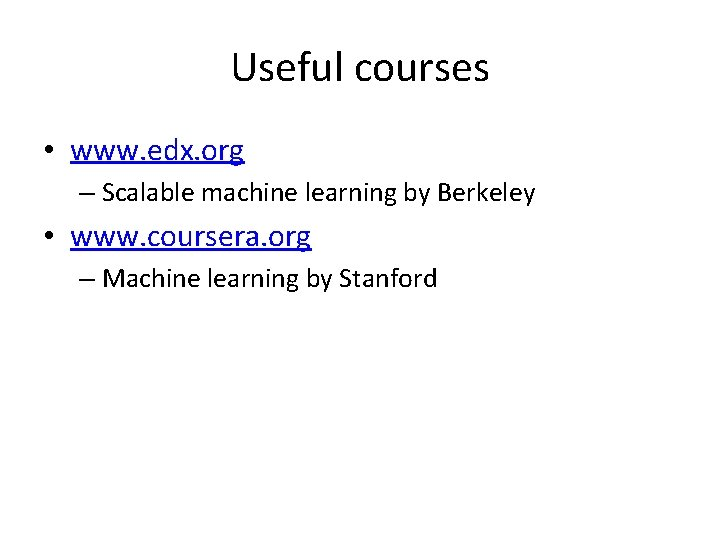Useful courses • www. edx. org – Scalable machine learning by Berkeley • www. coursera. org – Machine learning by Stanford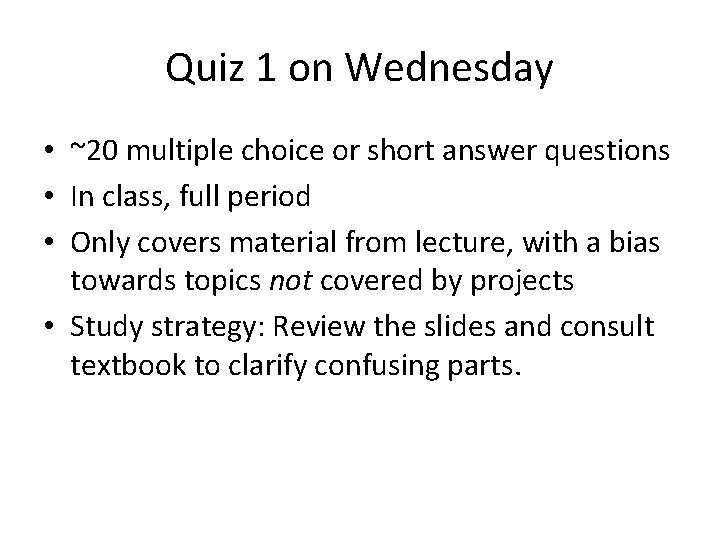Quiz 1 on Wednesday • ~20 multiple choice or short answer questions • In class, full period • Only covers material from lecture, with a bias towards topics not covered by projects • Study strategy: Review the slides and consult textbook to clarify confusing parts.Project 3 preview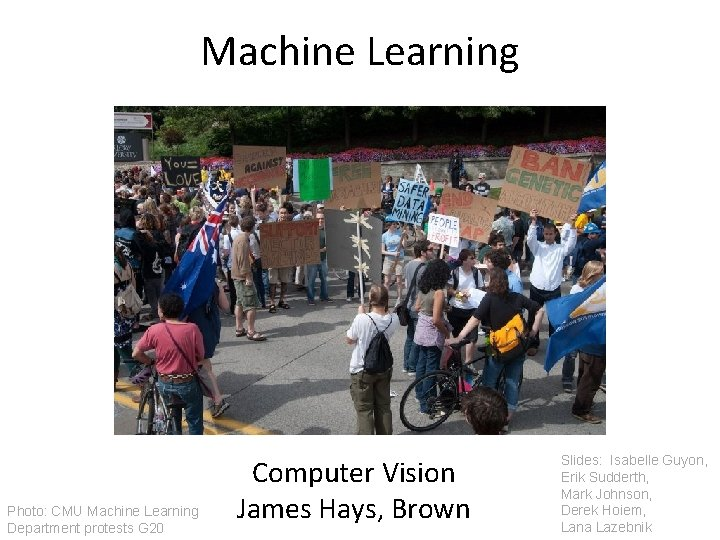Machine Learning Photo: CMU Machine Learning Department protests G 20 Computer Vision James Hays, Brown Slides: Isabelle Guyon, Erik Sudderth, Mark Johnson, Derek Hoiem, Lana Lazebnik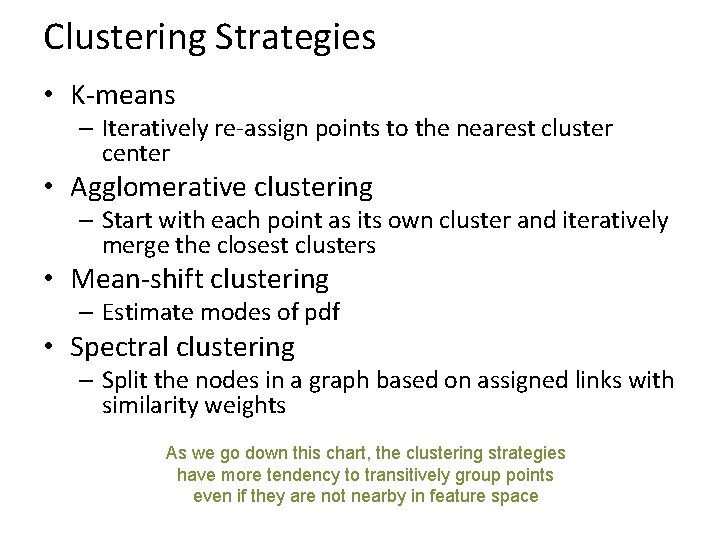Clustering Strategies • K-means – Iteratively re-assign points to the nearest cluster center • Agglomerative clustering – Start with each point as its own cluster and iteratively merge the closest clusters • Mean-shift clustering – Estimate modes of pdf • Spectral clustering – Split the nodes in a graph based on assigned links with similarity weights As we go down this chart, the clustering strategies have more tendency to transitively group points even if they are not nearby in feature space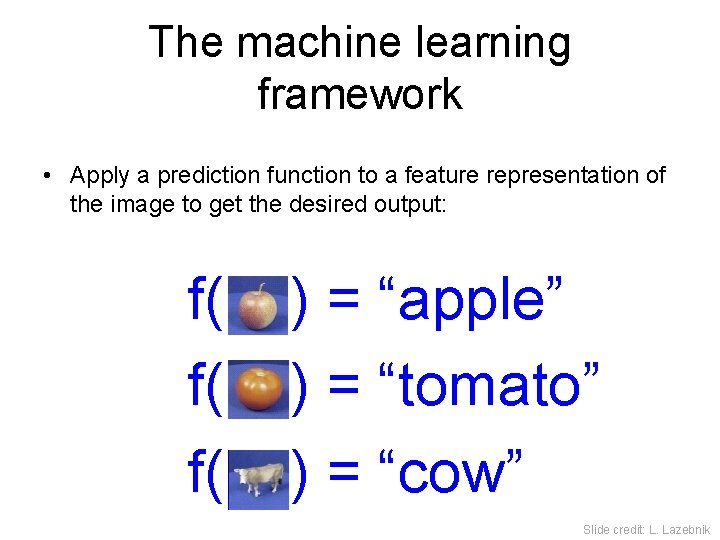The machine learning framework • Apply a prediction function to a feature representation of the image to get the desired output: f( f( f( ) = “apple” ) = “tomato” ) = “cow” Slide credit: L. LazebnikThe machine learning framework y = f(x) output prediction function Image feature • Training: given a training set of labeled examples {(x 1, y 1), …, (x. N, y. N)}, estimate the prediction function f by minimizing the prediction error on the training set • Testing: apply f to a never before seen test example x and output the predicted value y = f(x) Slide credit: L. LazebnikSteps Training Labels Training Images Image Features Training Learned model Prediction Testing Image Features Test Image Slide credit: D. Hoiem and L. Lazebnik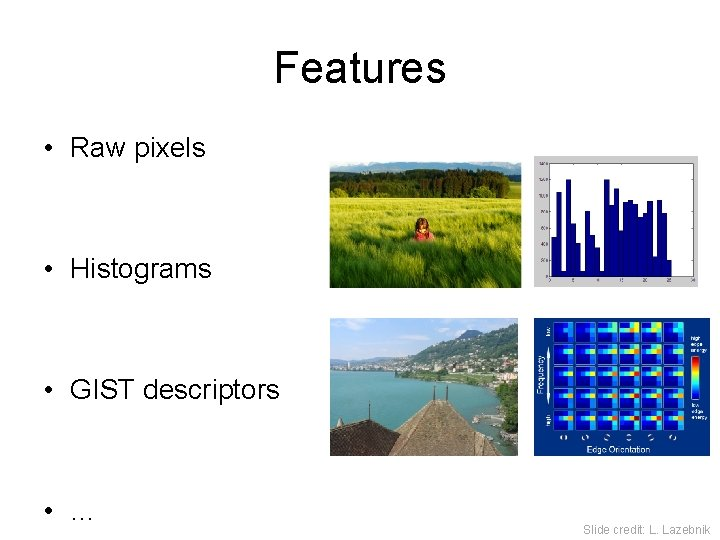Features • Raw pixels • Histograms • GIST descriptors • … Slide credit: L. LazebnikClassifiers: Nearest neighbor Training examples from class 1 Test example Training examples from class 2 f(x) = label of the training example nearest to x • All we need is a distance function for our inputs • No training required! Slide credit: L. Lazebnik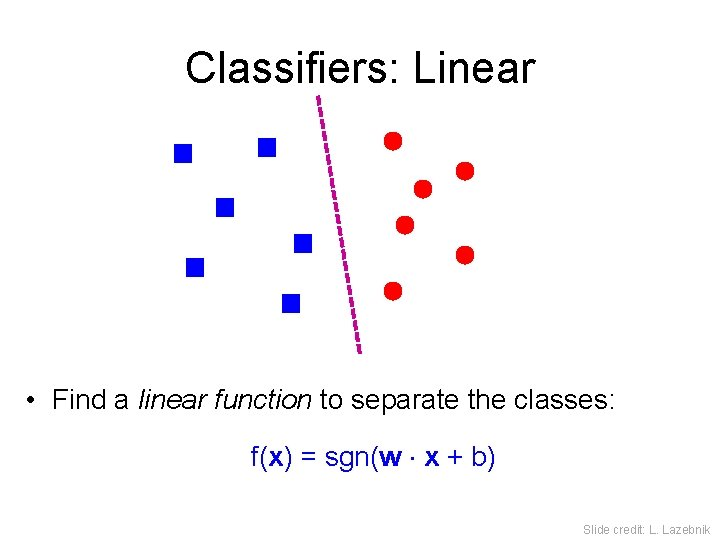Classifiers: Linear • Find a linear function to separate the classes: f(x) = sgn(w x + b) Slide credit: L. Lazebnik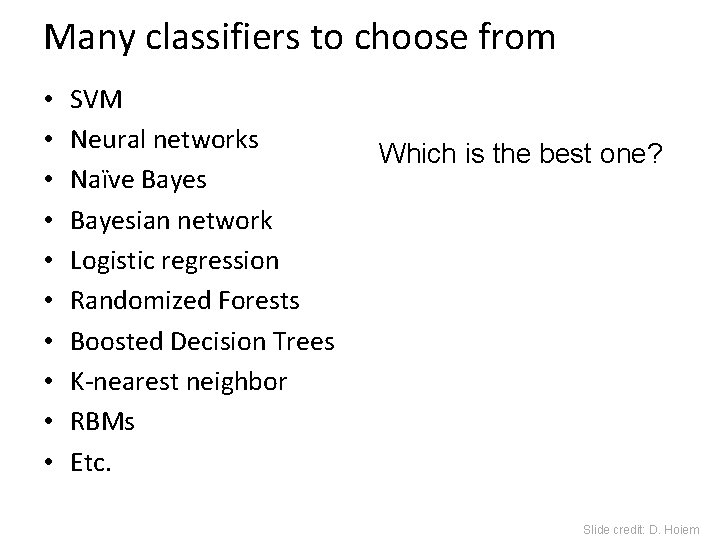Many classifiers to choose from • • • SVM Neural networks Naïve Bayesian network Logistic regression Randomized Forests Boosted Decision Trees K-nearest neighbor RBMs Etc. Which is the best one? Slide credit: D. HoiemRecognition task and supervision • Images in the training set must be annotated with the “correct answer” that the model is expected to produce Contains a motorbike Slide credit: L. LazebnikUnsupervised “Weakly” supervised Fully supervised Definition depends on task Slide credit: L. Lazebnik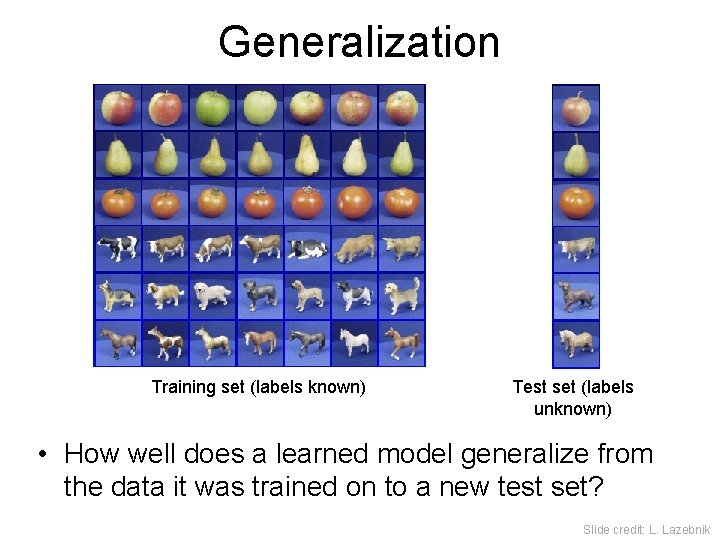Generalization Training set (labels known) Test set (labels unknown) • How well does a learned model generalize from the data it was trained on to a new test set? Slide credit: L. Lazebnik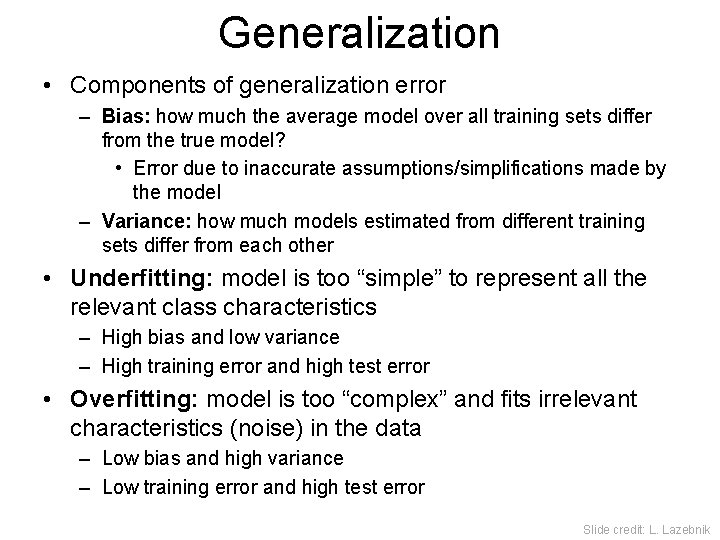Generalization • Components of generalization error – Bias: how much the average model over all training sets differ from the true model? • Error due to inaccurate assumptions/simplifications made by the model – Variance: how much models estimated from different training sets differ from each other • Underfitting: model is too “simple” to represent all the relevant class characteristics – High bias and low variance – High training error and high test error • Overfitting: model is too “complex” and fits irrelevant characteristics (noise) in the data – Low bias and high variance – Low training error and high test error Slide credit: L. Lazebnik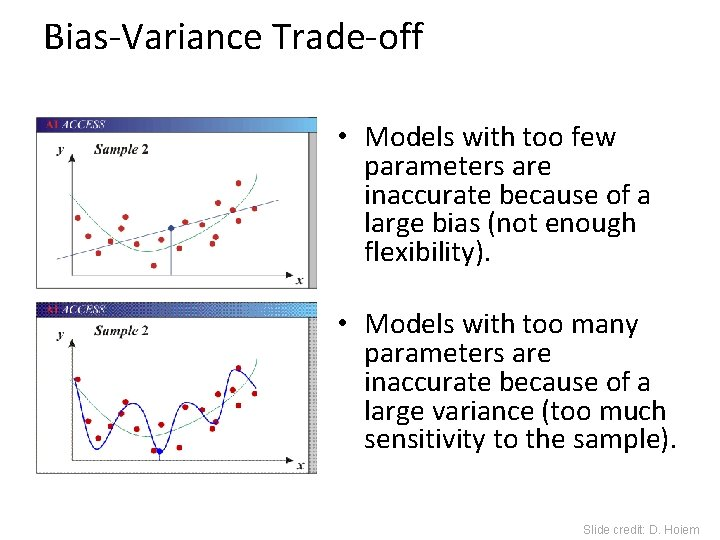Bias-Variance Trade-off • Models with too few parameters are inaccurate because of a large bias (not enough flexibility). • Models with too many parameters are inaccurate because of a large variance (too much sensitivity to the sample). Slide credit: D. HoiemBias-Variance Trade-off E(MSE) = noise 2 + bias 2 + variance Unavoidable error Error due to incorrect assumptions Error due to variance of training samples See the following for explanations of bias-variance (also Bishop’s “Neural Networks” book): • http: //www. inf. ed. ac. uk/teaching/courses/mlsc/Notes/Lecture 4/Bias. Variance. pdf Slide credit: D. Hoiem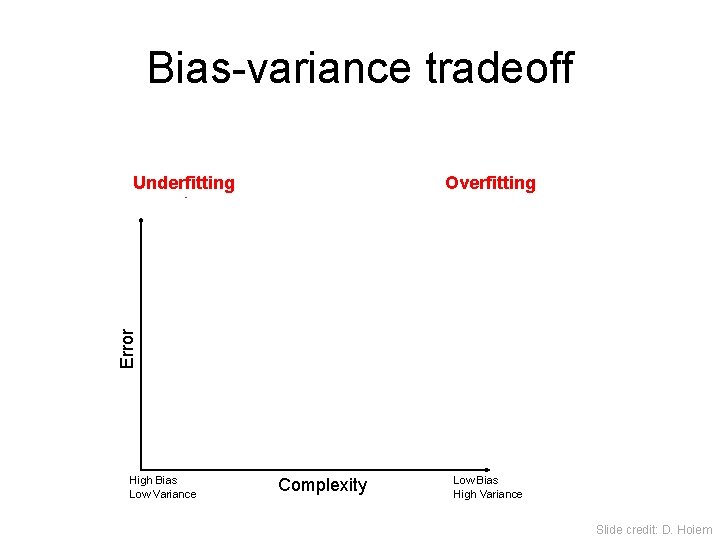Bias-variance tradeoff Overfitting Error Underfitting Test error Training error High Bias Low Variance Complexity Low Bias High Variance Slide credit: D. HoiemBias-variance tradeoff Test Error Few training examples High Bias Low Variance Many training examples Complexity Low Bias High Variance Slide credit: D. Hoiem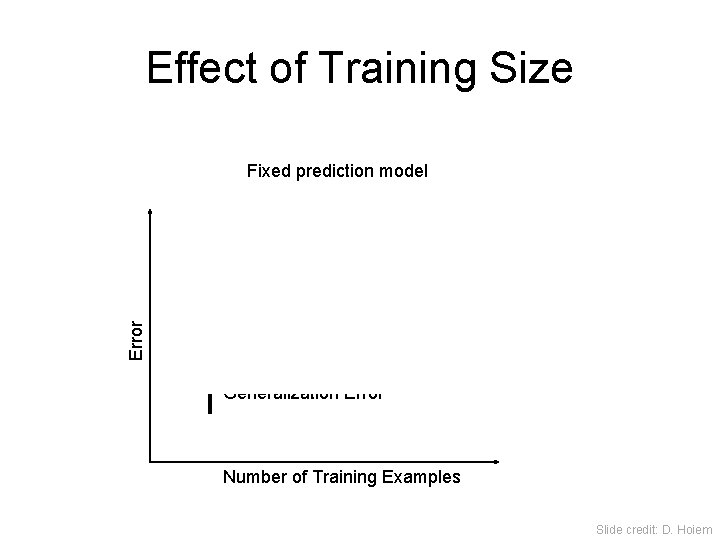Effect of Training Size Error Fixed prediction model Testing Generalization Error Training Number of Training Examples Slide credit: D. HoiemRemember… • No classifier is inherently better than any other: you need to make assumptions to generalize • Three kinds of error – Inherent: unavoidable – Bias: due to over-simplifications – Variance: due to inability to perfectly estimate parameters from limited data Slide credit: D. D. Hoiem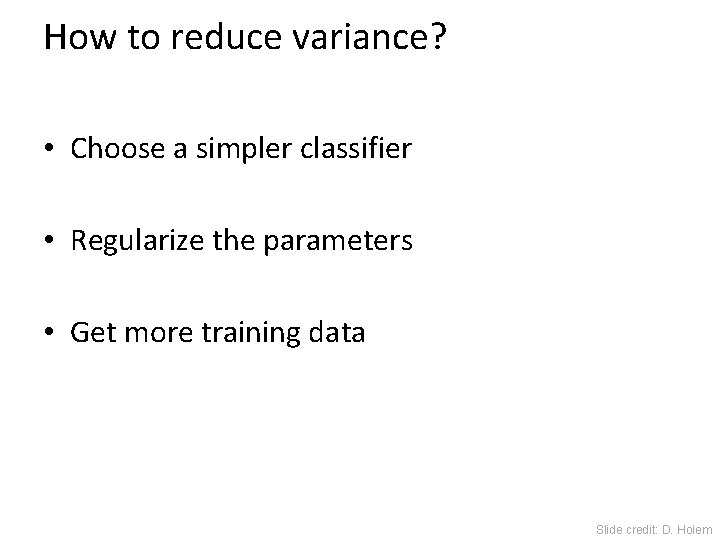How to reduce variance? • Choose a simpler classifier • Regularize the parameters • Get more training data Slide credit: D. HoiemVery brief tour of some classifiers • • • K-nearest neighbor SVM Boosted Decision Trees Neural networks Naïve Bayesian network Logistic regression Randomized Forests RBMs Etc.Generative vs. Discriminative Classifiers Generative Models Discriminative Models • Represent both the data and • Learn to directly predict the labels from the data • Often, makes use of • Often, assume a simple conditional independence boundary (e. g. , linear) and priors • Examples – Logistic regression – Naïve Bayes classifier – Bayesian network – SVM – Boosted decision trees • Models of data may apply to • Often easier to predict a label from the data than to future prediction problems model the data Slide credit: D. Hoiem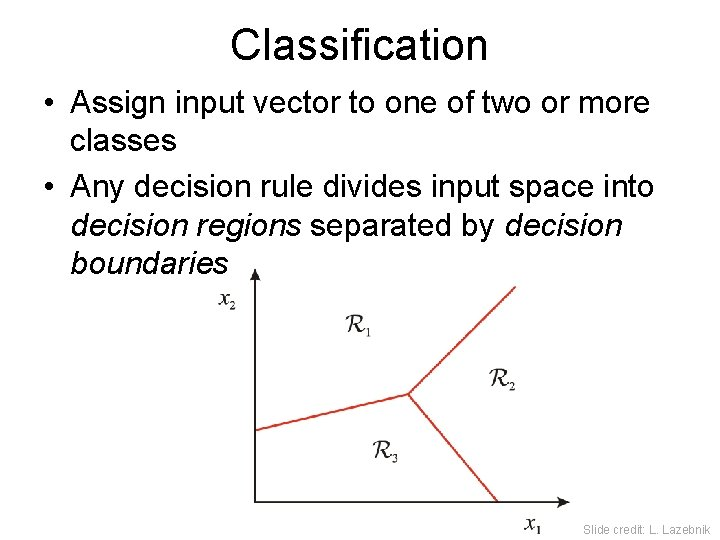Classification • Assign input vector to one of two or more classes • Any decision rule divides input space into decision regions separated by decision boundaries Slide credit: L. Lazebnik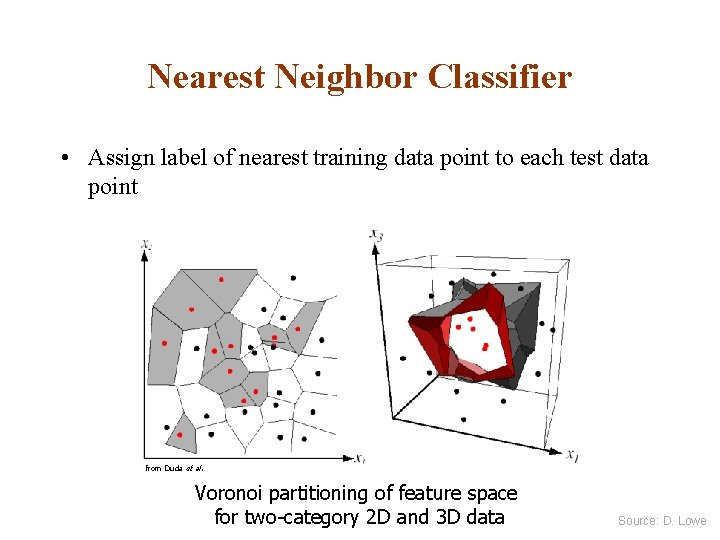Nearest Neighbor Classifier • Assign label of nearest training data point to each test data point from Duda et al. Voronoi partitioning of feature space for two-category 2 D and 3 D data Source: D. Lowe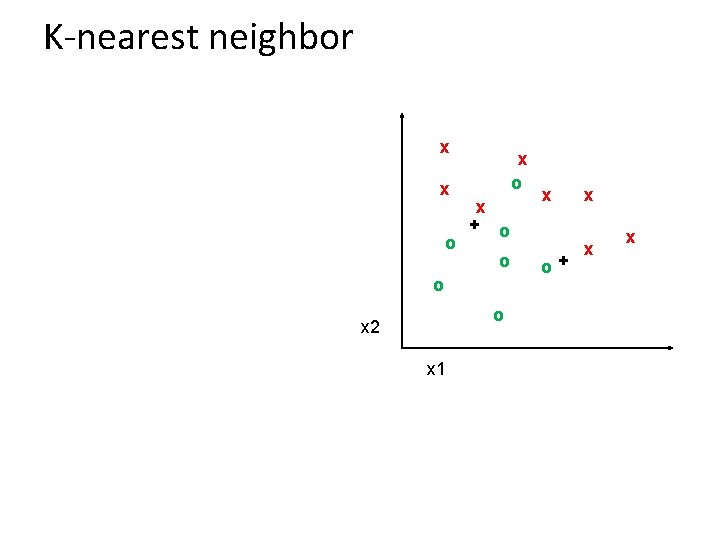K-nearest neighbor x x o x + o o x 2 x 1 x o+ x x x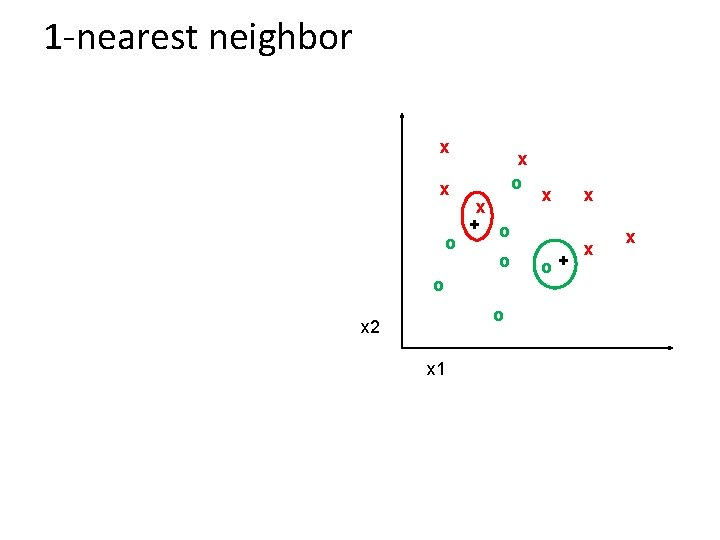1 -nearest neighbor x x o x + o o x 2 x 1 x o+ x x x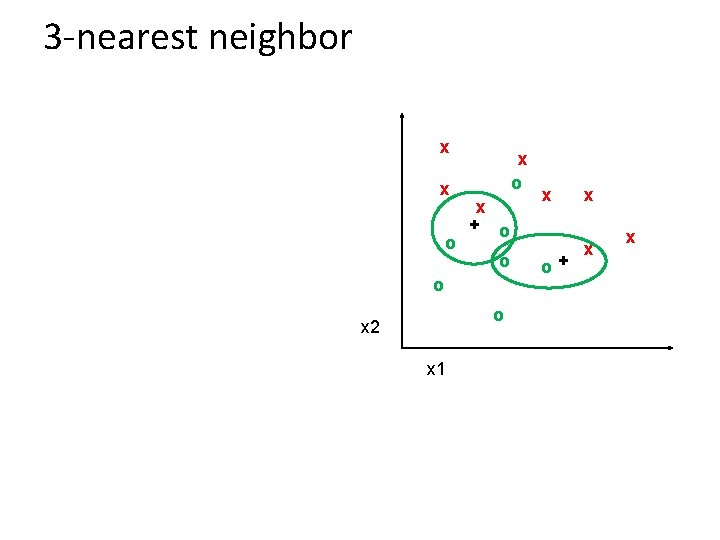3 -nearest neighbor x x o x + o o x 2 x 1 x o+ x x x5 -nearest neighbor x x o x + o o x 2 x 1 x o+ x x x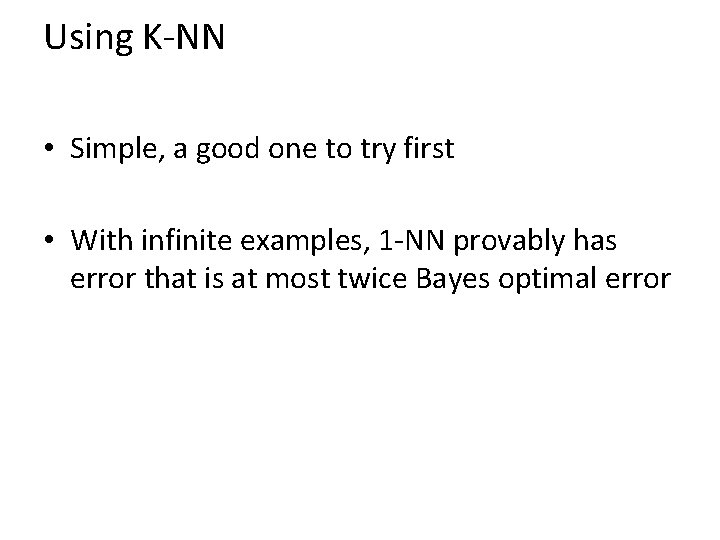Using K-NN • Simple, a good one to try first • With infinite examples, 1 -NN provably has error that is at most twice Bayes optimal error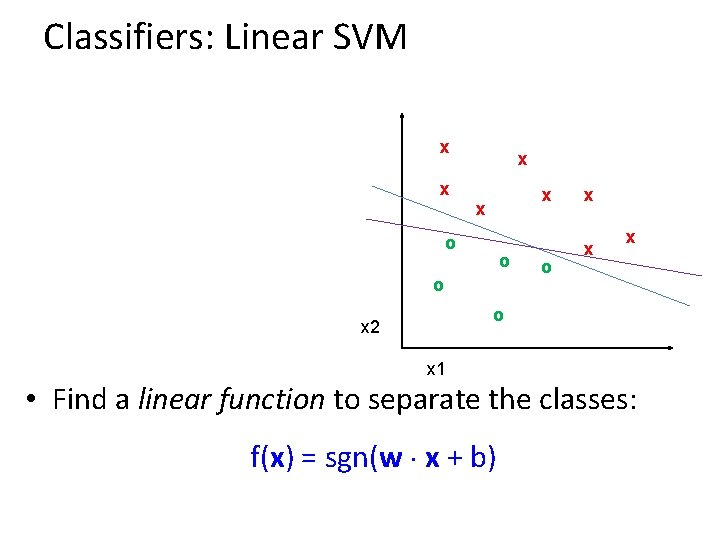Classifiers: Linear SVM x x x o o x x x o x 2 x 1 • Find a linear function to separate the classes: f(x) = sgn(w x + b)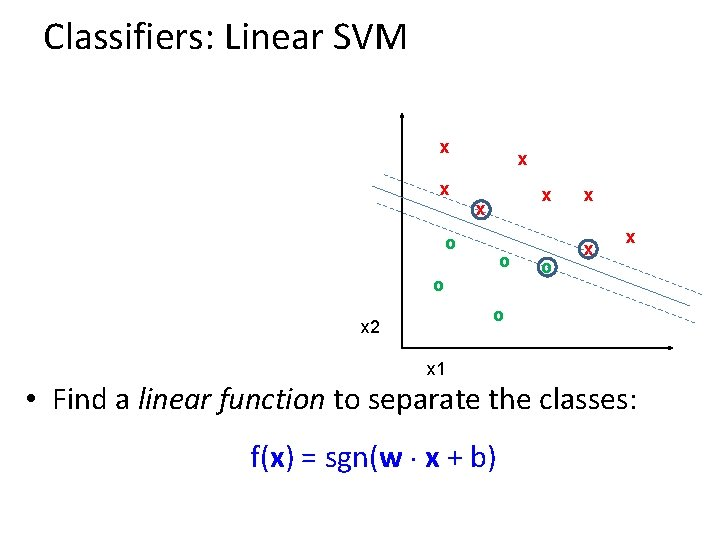Classifiers: Linear SVM x x x o o x x x o x 2 x 1 • Find a linear function to separate the classes: f(x) = sgn(w x + b)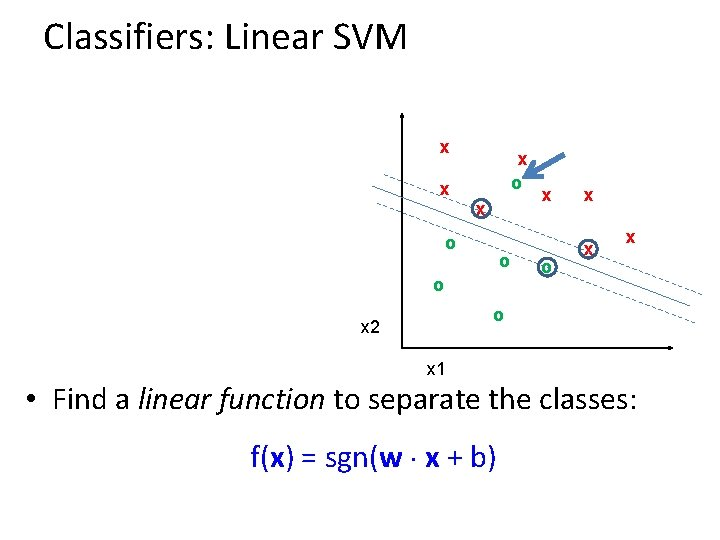Classifiers: Linear SVM x x x o o o x x x o x 2 x 1 • Find a linear function to separate the classes: f(x) = sgn(w x + b)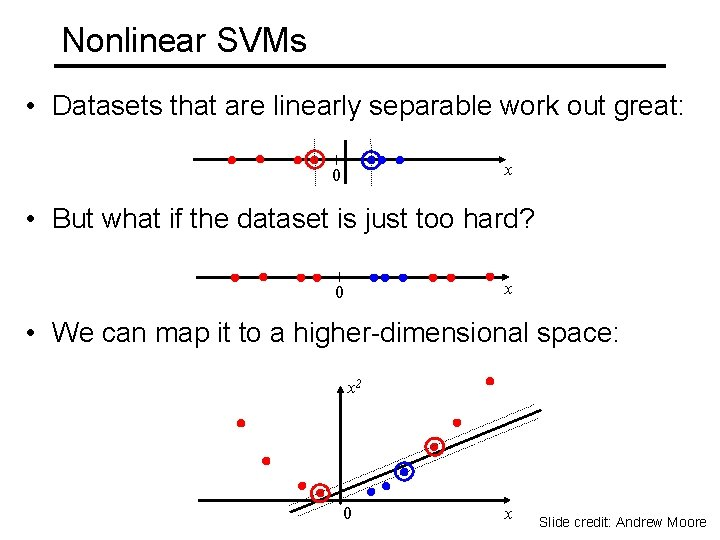Nonlinear SVMs • Datasets that are linearly separable work out great: x 0 • But what if the dataset is just too hard? x 0 • We can map it to a higher-dimensional space: x 2 0 x Slide credit: Andrew Moore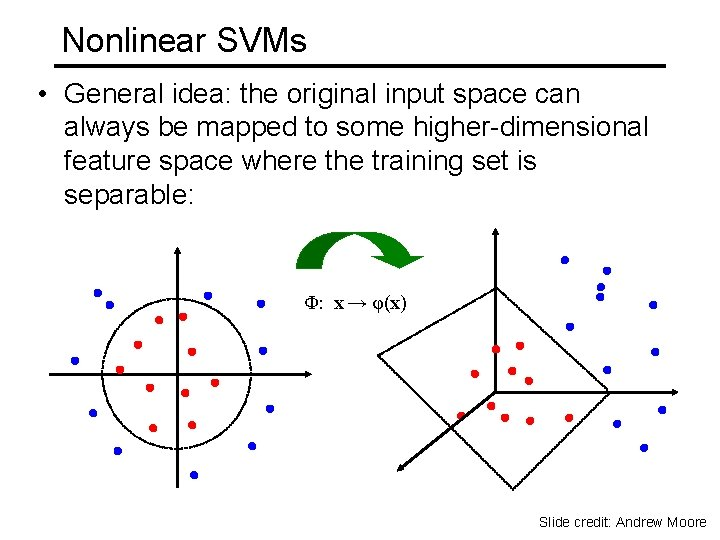Nonlinear SVMs • General idea: the original input space can always be mapped to some higher-dimensional feature space where the training set is separable: Φ: x → φ(x) Slide credit: Andrew MooreNonlinear SVMs • The kernel trick: instead of explicitly computing the lifting transformation φ(x), define a kernel function K such that K(xi , xj) = φ(xi ) · φ(xj) (to be valid, the kernel function must satisfy Mercer’s condition) • This gives a nonlinear decision boundary in the original feature space: C. Burges, A Tutorial on Support Vector Machines for Pattern Recognition, Data Mining and Knowledge Discovery, 1998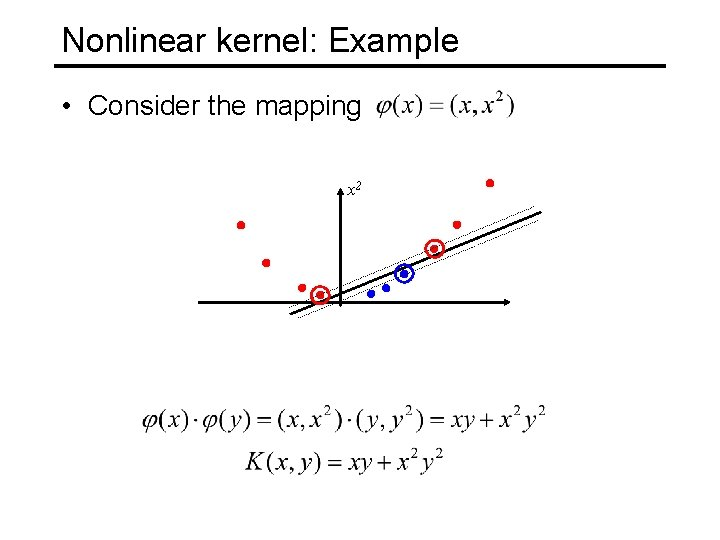Nonlinear kernel: Example • Consider the mapping x 2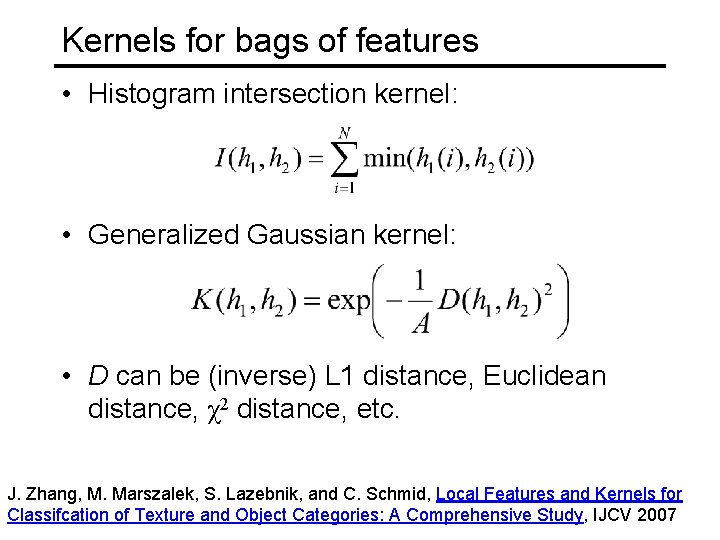Kernels for bags of features • Histogram intersection kernel: • Generalized Gaussian kernel: • D can be (inverse) L 1 distance, Euclidean distance, χ2 distance, etc. J. Zhang, M. Marszalek, S. Lazebnik, and C. Schmid, Local Features and Kernels for Classifcation of Texture and Object Categories: A Comprehensive Study, IJCV 2007Summary: SVMs for image classification 1. Pick an image representation (in our case, bag of features) 2. Pick a kernel function for that representation 3. Compute the matrix of kernel values between every pair of training examples 4. Feed the kernel matrix into your favorite SVM solver to obtain support vectors and weights 5. At test time: compute kernel values for your test example and each support vector, and combine them with the learned weights to get the value of the decision function Slide credit: L. Lazebnik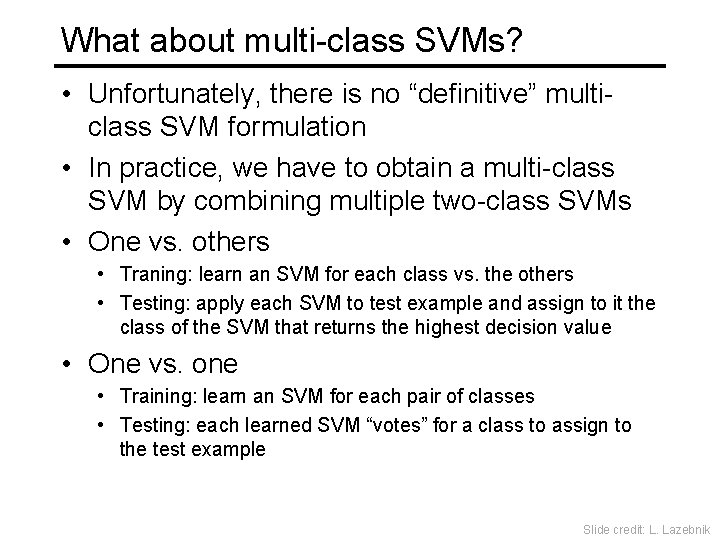What about multi-class SVMs? • Unfortunately, there is no “definitive” multiclass SVM formulation • In practice, we have to obtain a multi-class SVM by combining multiple two-class SVMs • One vs. others • Traning: learn an SVM for each class vs. the others • Testing: apply each SVM to test example and assign to it the class of the SVM that returns the highest decision value • One vs. one • Training: learn an SVM for each pair of classes • Testing: each learned SVM “votes” for a class to assign to the test example Slide credit: L. LazebnikSVMs: Pros and cons • Pros • Many publicly available SVM packages: http: //www. kernel-machines. org/software • Kernel-based framework is very powerful, flexible • SVMs work very well in practice, even with very small training sample sizes • Cons • No “direct” multi-class SVM, must combine two-class SVMs • Computation, memory – During training time, must compute matrix of kernel values for every pair of examples – Learning can take a very long time for large-scale problemsWhat to remember about classifiers • No free lunch: machine learning algorithms are tools, not dogmas • Try simple classifiers first • Better to have smart features and simple classifiers than simple features and smart classifiers • Use increasingly powerful classifiers with more training data (bias-variance tradeoff) Slide credit: D. HoiemSome Machine Learning References • General – Tom Mitchell, Machine Learning, Mc. Graw Hill, 1997 – Christopher Bishop, Neural Networks for Pattern Recognition, Oxford University Press, 1995 • Adaboost – Friedman, Hastie, and Tibshirani, “Additive logistic regression: a statistical view of boosting”, Annals of Statistics, 2000 • SVMs – http: //www. support-vector. net/icml-tutorial. pdf Slide credit: D. Hoiem